# Test: Sinusoidal Steady-State Analysis- 2

## 10 Questions MCQ Test Topicwise Question Bank for Electrical Engineering | Test: Sinusoidal Steady-State Analysis- 2

Description
Attempt Test: Sinusoidal Steady-State Analysis- 2 | 10 questions in 30 minutes | Mock test for Electrical Engineering (EE) preparation | Free important questions MCQ to study Topicwise Question Bank for Electrical Engineering for Electrical Engineering (EE) Exam | Download free PDF with solutions
QUESTION: 1

### The driving point admittance of the network shown below is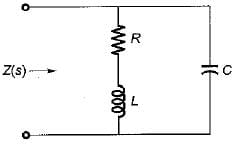Solution: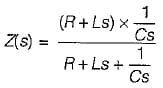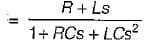∴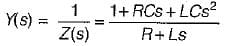QUESTION: 2

### Figure shown below represents a d.c. 10 V voltage source as well as a 10 V a.c. 50 Hz voltage source while Z1 and Z2 are two unknown impedances. When Z1 is connected across 10V d.c. source, it draws a current of 10 A while it draws a current of 8 A if connected across 10V a.c. source. Now, when Z2 is connected across 10 V d.c. source, it does not draw any current while it draws a current of 5 A if connected across 10 V a.c. source.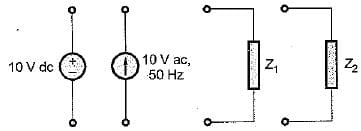Q. What are the elements in Z1 and Z2?

Solution:

When Z1 is connected across d.c. source, it draws 10 A current.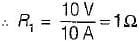Again, when Z1 is connected across a.c. source, it draws 8 A.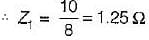Thus,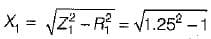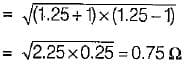Thus, Z1 = (1 + j0.75) Ω
Now, when Z2 is connected across 10 V d.c. source, it doesn’t draw any current. Hence, Z2 must be a capacitor which wilt act as open circuit for d.c. source.
Also, Z2 draws 5 A when connected to 10 V a.c. source.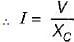or,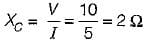Thus, Z2 = -j2 Ω

QUESTION: 3

### The current through the capacitor in the given circuit is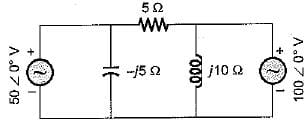Solution:

Since two voltage sources are present, we apply. Superposition theorem to find current through the capacitor i.e. current through -j5 Ω, reactance.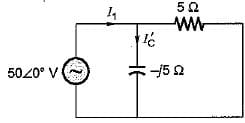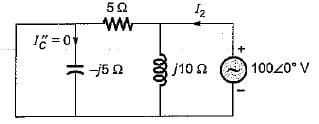Here,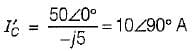and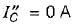So,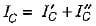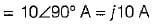QUESTION: 4

A parallel RLC circuit is said to be underdamped when

Solution:

The characteristic equation of a parallel RLC circuit is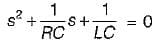Also,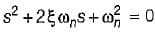Thus,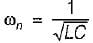and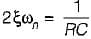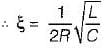for underdamped circuit, ξ < 1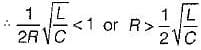QUESTION: 5

Assertion (A): A coil, when connected across 230 V dc supply, will draw more current in comparison to that when connected across 230 V ac supply.
Reason (R): The inductance of the coil opposes the flow of alternating current (not that of direct current).

Solution:
QUESTION: 6

A two terminal black box contains one of the R-L-C elements. When the black box is connected to a 220 V ac supply, the current through the source is I. When a capacitance of 0.1 F is inserted in series between the source and the box, the current through the source is 2I. The element is

Solution:

As current is increased therefore, new impedance should reduce. If the unknown element will be inductive, the new impedance will be (XL- XC) Ω, so that the new value of current will increase.

QUESTION: 7

A system function has a pole at s = 0 and zero at s = -1. The constant multiplier is unity. For an excitation cost, the steady state response is given by

Solution: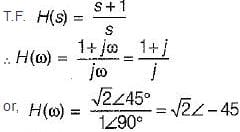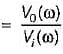Thus,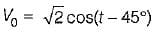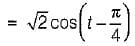QUESTION: 8

For the a.c. circuit given below, what is the value of I?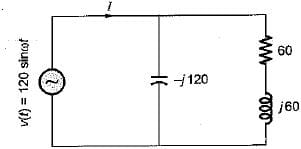Solution: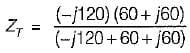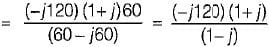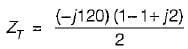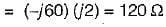So,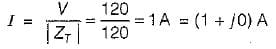QUESTION: 9

Consider the following pole-zero diagram of a system,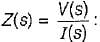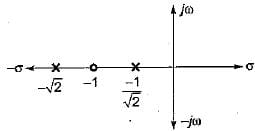What will be the magnitude of the voltage phasor for i(t) = sint?

Solution:

From the pole-zero plot,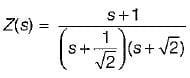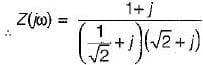(Since sint = i(t); ω = 1 rad/s)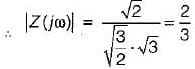so,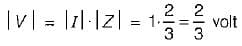QUESTION: 10

A coil takes a current of 1∠60° A (lag) from a 100 V, 50 Hz supply. The resistance of the coil is

Solution: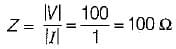Also,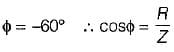or, cos 60° = R/100
or, R = 100 cos 60°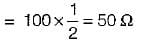Use Code STAYHOME200 and get INR 200 additional OFF Use Coupon Code